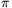Mathematical and Physical Journal
for High Schools
Issued by the MATFUND Foundation
 Already signed up? New to KöMaL?

#Problem A. 546. (November 2011)

A. 546. Show thatfor every positive integer k.

(5 pont)

Deadline expired on December 12, 2011.

Solution 1. Let Un(x) be the nth Chebyshev-polynomial of the second kind, for which Un(cos t)sin t=sin ((n+1)t). The roots of U2k are the numbers,, ...,and their negatives, xk+1=-x+1, xk+2=-x2, ..., x2k=-xk. On the left-hand side of the statement we have the sum of the squares of these numbers; the statement is equivalent with(1)

Lemma 1. U0(x)=1, U2(x)=4x2-1, and U2k+2(x)+U2k-2(x)=(4x2-2)U2k(x) for k1.

Proof. From U0(cos t)sin t=sin t, we get U0(x)=1. Similarly, from U2(cos t)sin t=sin (3t)=(4cos2-1)sin t we get U2(x)=4x2-1.

From the identity sin (a+b)+sin (a-b)=2sin acos b we obtain

U2k+2(cos t)sin t+U2k-2(cos t)sin t=

=sin ((2k+3)t)+sin ((2k-1)t)=2sin ((2k+1)t)cos (2t)=

=2U2k(cos t)sin t.(2cos2-1),

so U2k+2(x)+U2k-2(x)=(4x2-2)U2k(x).

Lemma 2. Let. Then ak,0=(-1)k, ak,1=0 és ak,2=(-1)k+1.2k(k+1).

Proof. Apply induction on k. If k=0 then U0(x)=1, so a0,0=1=(-1)k, a0,1=0, and a0,2=0=-4k(k+1) If k=1 then U0(x)=4x2-1, so a1,0=-1=(-1)k, a1,1=0, and a1,2=4=2k(k+1). Hence, the lemma holds for k=0 and k=1.

For the induction step assume the lemma for k and (k-1). By Lemma 1,ak+1,0=-2.(-1)k-(-1)k-1=(-1)k+1,

ak+1,1=0,

finally

ak+1,2=4.(-1)k-2.(-1)k+1.2k(k+1)-(-1)k.2k(k-1)=

=(-1)k(4+4k(k+1)-2k(k-1))=(-1)k+2.2(k+1)(k+2).

The lemma is proved.

Now from the Viéte formulae we findSolution 2. Let, thenegész számra.

From the identitywe havesoApplyingagain,Therefore,Solution 3. We use U2k again and prove (1). The polynomial can be factorized as, where c is the leading coefficient. ThenandSubstituting x=0 we obtain(2)

We compute the values U2k(0), U2k'(0), U2k''(0) by differentiating

 U2k(cos t)sin t=sin ((2k+1)t) (3a)

twice.

 -U2k'(cos t)sin2t+U2k(cos t)cos t=(2k+1)cos ((2k+1)t), (3b)
 U2k''(cos t)sin3t-3U2k'(cos t)sin tcos t-U2k(cos t)sin t=-(2k+1)2sin ((2k+1)t). (3c)

By substituting t=/2 in (3a--3c) we get U2k(0)=(-1)k and U2k'(0)=0, furthermore

U2k''(0)=(-1)k-(-1)k.(2k+1)2=(-1)k+1.4k(k+1).

Plugging these into (2),### Statistics:

 7 students sent a solution. 5 points: Ágoston Tamás, Gyarmati Máté, Janzer Olivér, Kovács 444 Áron, Mester Márton, Omer Cerrahoglu, Szabó 928 Attila.

Problems in Mathematics of KöMaL, November 2011